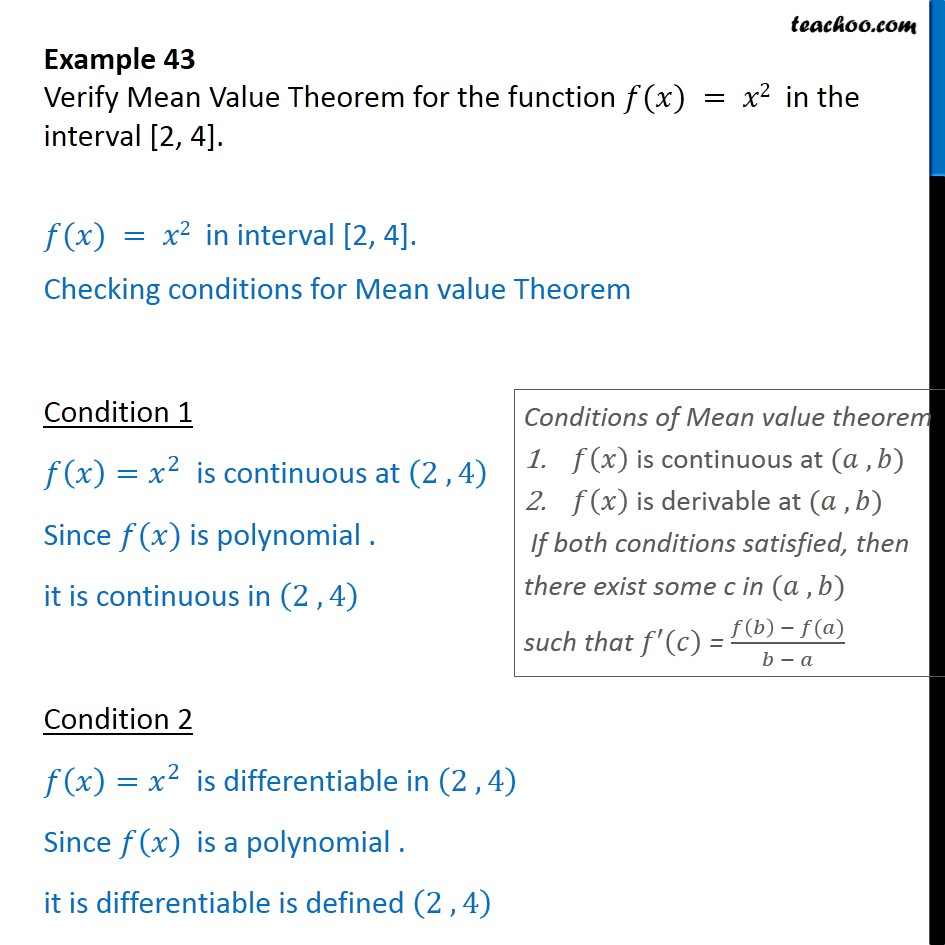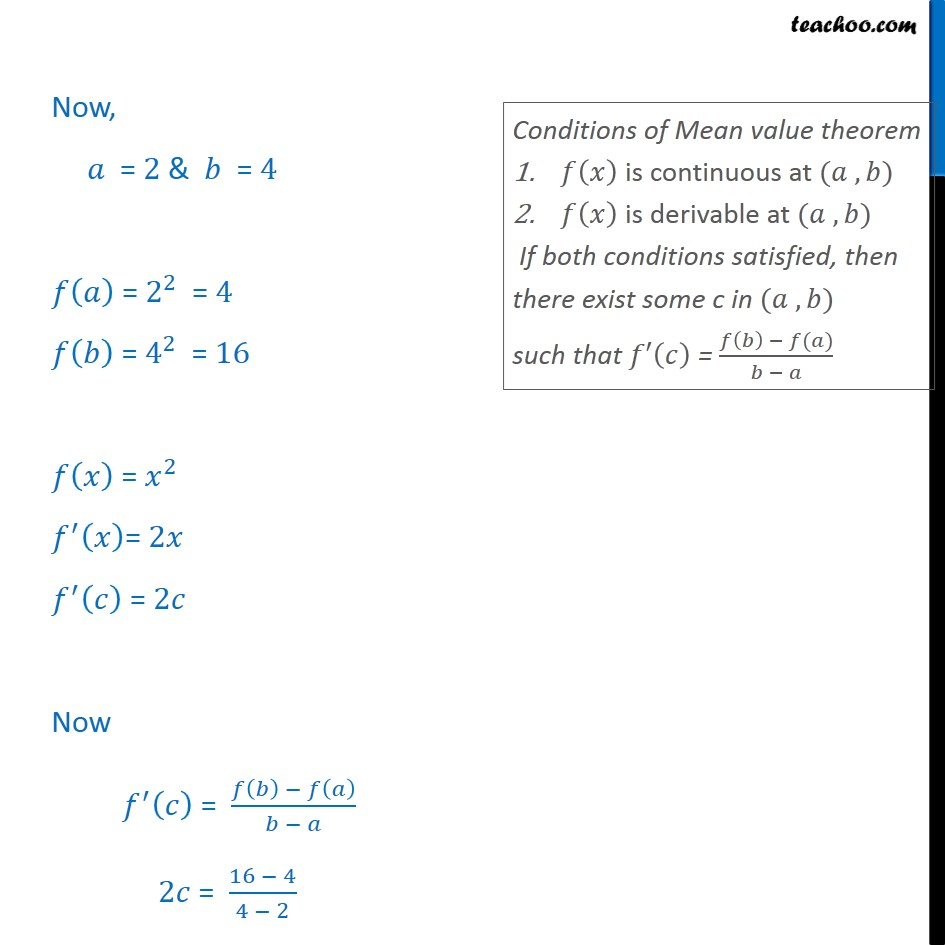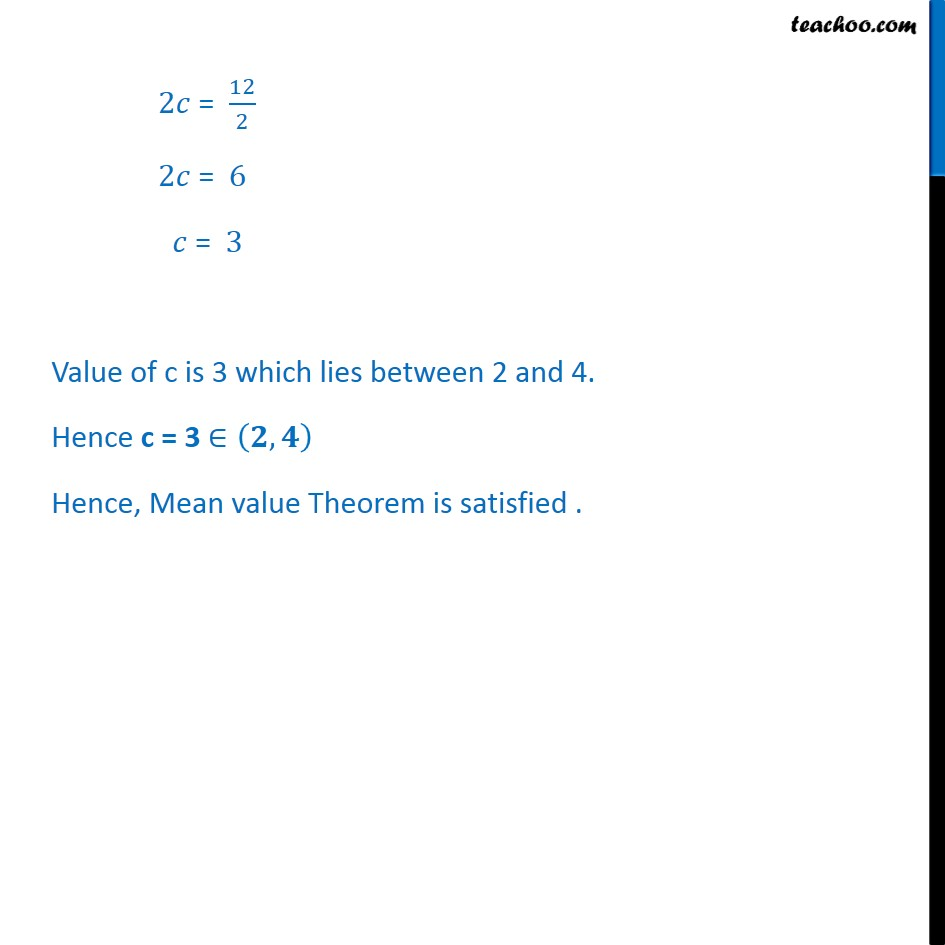1. Chapter 5 Class 12 Continuity and Differentiability
2. Serial order wise
3. Examples

Transcript

Example 43 Verify Mean Value Theorem for the function ( ) = 2 in the interval [2, 4]. ( ) = 2 in interval [2, 4]. Checking conditions for Mean value Theorem Condition 1 = 2 is continuous at 2 , 4 Since ( ) is polynomial . it is continuous in 2 , 4 Condition 2 = 2 is differentiable in 2 , 4 Since is a polynomial . it is differentiable is defined 2 , 4 Now, = 2 & = 4 = 2 2 = 4 = 4 2 = 16 = 2 = 2 = 2 Now = 2 = 16 4 4 2 2 = 12 2 2 = 6 = 3 Value of c is 3 which lies between 2 and 4. Hence c = 3 , Hence, Mean value Theorem is satisfied .

Examples

About the AuthorDavneet Singh
Davneet Singh is a graduate from Indian Institute of Technology, Kanpur. He has been teaching from the past 9 years. He provides courses for Maths and Science at Teachoo.